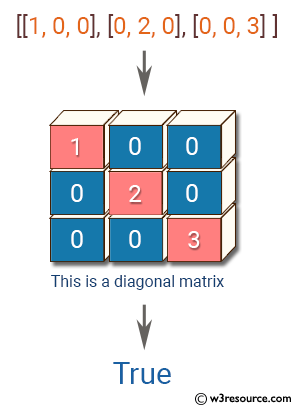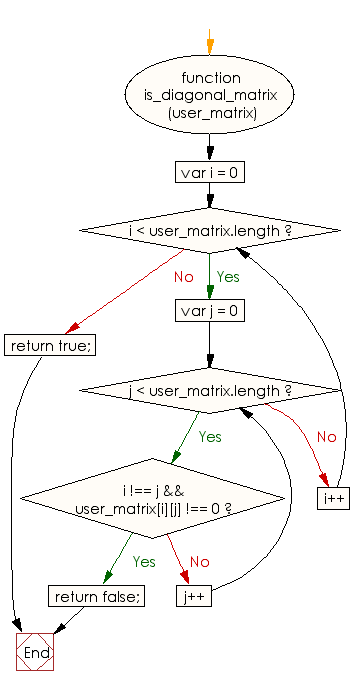# JavaScript: Check whether a matrix is a diagonal matrix or not

## JavaScript Basic: Exercise-115 with Solution

Write a JavaScript program to check whether a matrix is a diagonal matrix or not. In linear algebra, a diagonal matrix is a matrix in which the entries outside the main diagonal are all zero (the diagonal from the upper left to the lower right).

Example:
[1, 0, 0], [0, 2, 0], [0, 0, 3] ]) = true
[1, 0, 0], [0, 2, 3], [0, 0, 3] ]) = false

Pictorial Presentation:Sample Solution:

HTML Code:

``````<!DOCTYPE html>
<html>
<meta charset="utf-8">
<meta name="viewport" content="width=device-width">
<title> Check whether a matrix is a diagonal matrix or not</title>
<body>

</body>
</html>
```
```

JavaScript Code:

``````function is_diagonal_matrix(user_matrix) {
for (var i = 0; i < user_matrix.length; i++) {
for (var j = 0; j < user_matrix.length; j++) {
if (i !== j && user_matrix[i][j] !== 0)
return false;
}
}
return true;
}

console.log(is_diagonal_matrix([[1, 0, 0], [0, 2, 0], [0, 0, 3] ]));
console.log(is_diagonal_matrix([[1, 0, 0], [0, 2, 3], [0, 0, 3] ]));
``````

Sample Output:

```true
false
```

Flowchart:ES6 Version:

``````function is_diagonal_matrix(user_matrix) {
for (let i = 0; i < user_matrix.length; i++) {
for (let j = 0; j < user_matrix.length; j++) {
if (i !== j && user_matrix[i][j] !== 0)
return false;
}
}
return true;
}

console.log(is_diagonal_matrix([[1, 0, 0], [0, 2, 0], [0, 0, 3] ]));
console.log(is_diagonal_matrix([[1, 0, 0], [0, 2, 3], [0, 0, 3] ]));
``````

Live Demo:

See the Pen javascript-basic-exercise-115 by w3resource (@w3resource) on CodePen.

What is the difficulty level of this exercise?

Test your Programming skills with w3resource's quiz.

﻿

## JavaScript: Tips of the Day

Returns the sum of the powers of all the numbers from start to end (both inclusive)

Example:

```const sumPower = (end, power = 2, start = 1) =>
Array(end + 1 - start)
.fill(0)
.map((x, i) => (i + start) ** power)
.reduce((a, b) => a + b, 0);
console.log(sumPower(10)); // 385
console.log(sumPower(10, 3)); // 3025
console.log(sumPower(10, 3, 5)); // 2925
```

Output:

```385
3025
2925
```